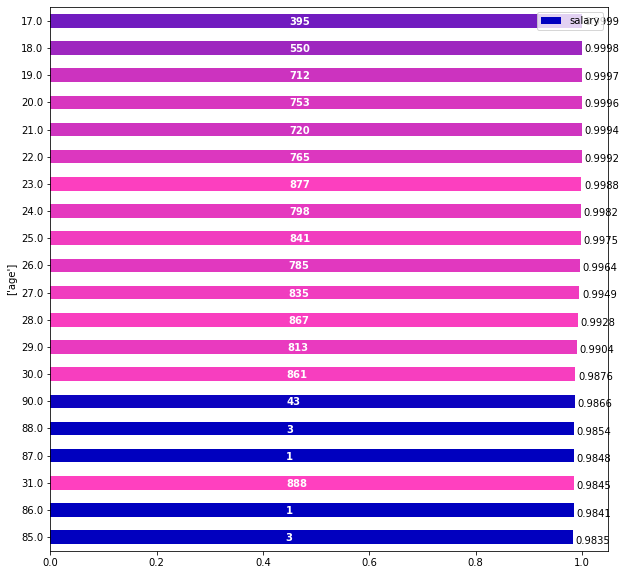This module calculates and plots waterfall chart, this entire module was made by Pavel (Pak)

First let's train a model to analyze

path = untar_data(URLs.ADULT_SAMPLE)

dep_var = 'salary'
cat_names = ['workclass', 'education', 'marital-status', 'occupation', 'relationship', 'race']
cont_names = ['age', 'fnlwgt', 'education-num']
procs = [Categorify, FillMissing, Normalize]

splits = IndexSplitter(list(range(800,1000)))(range_of(df))
to = TabularPandas(df, procs, cat_names, cont_names, y_names="salary", splits=splits)

learn = tabular_learner(dls, layers=[200,100], metrics=accuracy)
learn.fit(1, 1e-2)

epoch train_loss valid_loss accuracy time
0 0.368659 0.412912 0.810000 00:03

## classInterpretWaterfall[source]

InterpretWaterfall(learn, df, fields, sampl_row, max_row_used=None, use_log=False, use_int=False, num_tests=1) :: Interpret

How does this version calculate each columns part.

• Calculate mean prediction for all the dataset. It will be the starting point for price of an indivilual row to shift from
• For every column calculate the difference between this row prediction and a mean prediction of this column shuffled (how this particular column for a certain values in other columns shifts the dep_var and in what direction)
• Assume that sum of these differences can be transfered as forces onthe first meran predictions
• Plot these forces

This class allows you to calculate and plot Waterfall graph. Also in can be useful in determining and vizualizing what is the best value of particular feature for a given row of data. Calculate all the parameters to plot Waterfall graph for a sampl_row

• fields
  list of lists of columns to analyze, connected columns should be in the same list element (as a list)
• sampl_row
  row that is analyzed
• max_row_used
  how many rows to use for calculation. len(df) -- by default
Can be absolute value or coeffficient (from the len(df))
On big datasets can easily be set to lower values as it's enough data for calculating differences anyway. 10k rows is often enough
• num_tests
  is used to reduce memory consumption, each run uses max_row_used/num_tests rows, the more 'num_tests' the less memory consumption is
• use_log=True
  is needed if we have transformed depended variable into log
• use_int=True
  is needed if we want to log-detransformed (exponented) var to me integer not float
fields = cat_names+cont_names

cur_item = df.iloc

cur_item

age                           23
workclass                Private
fnlwgt                    529223
education              Bachelors
education-num                 13
marital-status     Never-married
occupation                   NaN
relationship           Own-child
race                       Black
sex                         Male
capital-gain                   0
capital-loss                   0
hours-per-week                10
native-country     United-States
salary                      <50k
Name: 10, dtype: object
fields = ['workclass', 'education', 'marital-status', 'occupation', 'relationship', 'race', 'age', 'fnlwgt', 'education-num']

cat_names+cont_names

['workclass', 'education', 'marital-status', 'occupation', 'relationship', 'race', 'education-num_na', 'age', 'fnlwgt', 'education-num']
wf = InterpretWaterfall(learn=learn, df=df, fields=fields,
sampl_row=cur_item, max_row_used=0.3)

wf.get_forces()

OrderedDict([('marital-status ( Never-married)', 0.03135448694229126),
('age (23)', 0.024528861045837402),
('relationship ( Own-child)', 0.007003426551818848),
('occupation (nan)', 0.0046416521072387695),
('race ( Black)', 0.0011506080627441406),
('workclass ( Private)', 0.0011272430419921875),
('fnlwgt (529223)', 0.001011967658996582),
('education ( Bachelors)', -3.4868717193603516e-05)])
wf.plot_forces()


Let's see what ages how affect this particular row

wf.plot_variants(fields=['age'])wf.get_variants_pd(fields=['age'])

feature salary times
38 17.0 0.999879 395.0
32 18.0 0.999821 550.0
27 19.0 0.999737 712.0
22 20.0 0.999613 753.0
26 21.0 0.999433 720.0
... ... ... ...
30 51.0 0.955168 595.0
29 50.0 0.954988 602.0
31 49.0 0.954858 577.0
28 47.0 0.954809 708.0
33 48.0 0.954788 543.0

73 rows × 3 columns

education andeducation-num are 100% correlated feature, we totally should group them

fields = ['workclass', ['education', 'education-num'], 'marital-status', 'occupation', 'relationship', 'race', 'age', 'fnlwgt']

wf = InterpretWaterfall(learn=learn, df=df, fields=fields,
sampl_row=cur_item, max_row_used=0.3)

wf.get_forces()

OrderedDict([('marital-status ( Never-married)', 0.03127652406692505),
('age (23)', 0.024258792400360107),
('relationship ( Own-child)', 0.006926894187927246),
('occupation (nan)', 0.004605710506439209),
('workclass ( Private)', 0.001170337200164795),
('race ( Black)', 0.0011529326438903809),
('fnlwgt (529223)', 0.001004636287689209),
('education ( Bachelors), education-num (13.0)',
-0.00014448165893554688)])
wf.plot_forces()


Methods exposed: plot_forces -- plot waterfall graph calculated in initialization, get_forces -- outputs all the forces for a given row as a ordered dict, plot_variants -- plot graph of different variants of values of a particular column